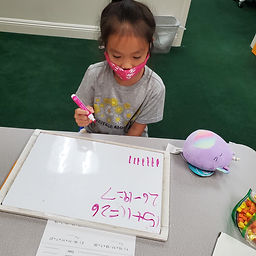## Ms. Raejean

### Target 1​

###### Lesson Type:

Continuation

Number Operation

:

Computation

Solve multi-step addition and subtraction problems within 50.

###### 1:

Solve problems that have more than one operation, addition and subtraction.

###### 2:

Understand when solving a problem that has both addition and subtraction operations that solving starts on the left and continues to the right.

2nd

###### Vocabulary:

Multi-step, Addition, Subtraction, Equation, Equal, Unknown Numbers

Activities:

Students continued to solve multi-step problems. Students solved problems from left to right, then solved for the unknown number. Students used tally marks and jumps on a number line to add and subtract. We discussed how it's easier to count up rather than count back. Ex. 26 - 19: Start with 19 and count up to 26 vs. count back from 26 to 19.### Home Exploration

###### Guiding Questions:## Absent Students:

### Target 2

:

###### 1:

Study a given arithmetic sequence to determine what rule explains the sequence (i.e., 2-4-6-8-10…each number is the sequence gets bigger by 2).

###### 2:

Write an arithmetic rule to explain a given sequence.

###### 3:

Understand that a pattern can be described by stating the rule that it is following.

2nd

###### Vocabulary:

Sequence, Pattern, Rule

Activities:

Students continued to identify the pattern and determine the next number in the sequence. Cubes were used to physically see the pattern to figure out how it changed from one group to the next. Students could see if the the pattern grew or got smaller, and they could count how many cubes were added or taken away.### Home Exploration

###### Guiding Questions:### Target 3

:

###### Vocabulary:

Activities:### Home Exploration